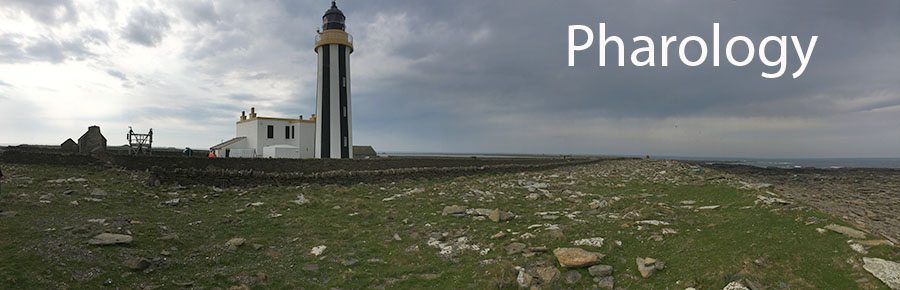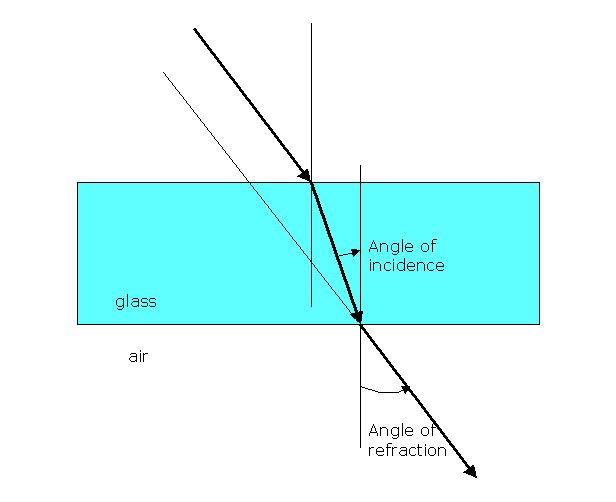## The World Lighthouse Hub

Pharology - How Lighthouses Work - H11

## H11: Refraction

Q: What is Refraction?

Refraction occurs when light passes from one medium to another. Refraction is a change in direction of the path of the light ray and this is brought about by the interaction of the light with the medium which may lead to some absorption of the the light and causes a change in its speed. The size of this interaction is defined by a property of the medium called the refractive index.

In the diagram below, you will notice that the ray of light is bent towards the normal (or perpendicular), that is, the angle of incidence is greater than the angle of refraction. This is because the refractive index of glass (crown glass can take values between 1.48 and 1.6) is greater than the refractive index of air (1.0). Perspex has a refractive index of 1.495 and polystyrene has a value of 1.591.

If we calculate the ratio of sin(angle of incidence) / sin(angle of refraction) we obtain the constant that we call the refractive index. This is called Snell's Law. By inspection, you can see that the greater the amount of refraction (deviation from the original path) the greater is the value of refractive index.

It is important to take this argument a stage further as you will see in a moment.

Consider that the ray has now passed through the glass and reaches the back face - we have drawn the glass as a rectangular block with a back face parallel to the front face. Exactly the same arguments apply that were used above: all the light may leave the medium, all of it may not leave the medium, or just some of it may leave. Let's consider that all of it leaves the glass. The light ray is refracted, as before, but now it is entering a medium (air) with smaller refractive index so the angle of refraction is now greater than the angle of incidence. Because the situation is an exact reversal of the first, the light emerges from the glass along a line parallel to the path it original took through the air, but it has been shifted laterally (sideways) by a small amount.

The design of optics in lighthouses uses all these properties to both reflect and refract light using glass of different sizes and shapes so as to obtain concentrated bright beams of light from light sources. A system that uses refraction only is called dioptric, whilst a system that uses reflection only is called catoptric. A system that uses both reflection and refraction, as most modern optics do, is called catadioptric.Figure H11.1: A diagram to show how a ray of light travelling in air can enter glass and be diverted from its original path.Figure H11.2: The diversion is reversed upon exit of the light ray from glass into air, provided that the two glass edges are parallel.